# How the Electric Power Grids Function

## Power Grid

What is a power grid?

The power grid forms a bridge between electrical suppliers and consumers through interconnected networks.

The electrical power grid consists of three main parts:

1. Generating plant for electric power.
2. Transmission of the electric power.
3. Distribution of the electric power.

## Electrical Power Generation in Power Stations

Normally, electric power generating plants are located near the source of power generation like dams, coal mines, etc. They may be in remote locations, and the generated power has to be supplied to the consumer in the city.

Sources for Power Generation:

The following types of resources are available for generating electrical energy for distribution:

• Conventional Methods

1.Thermal:

Thermal energy or Nuclear Energy used for producing steam for turbines which will drive the alternators (rotating AC generators).

2. Hydro-Electric:

Potential of water stored at higher altitudes is utilized as it is passes through water turbines which drive the alternators.

• Non- Conventional Methods:

1. Wind Power:

High velocities of wind are utilized in driving wind turbines coupled to alternators.

## Sources of Power Generation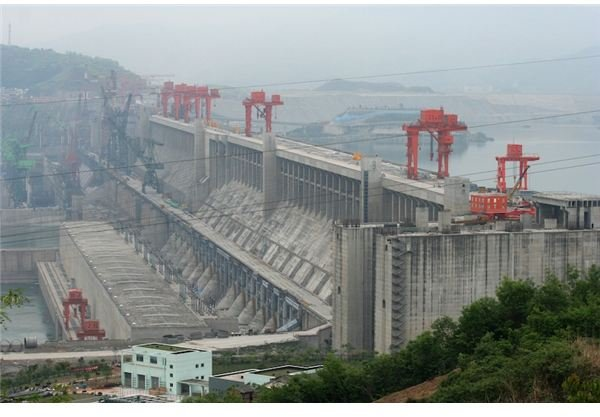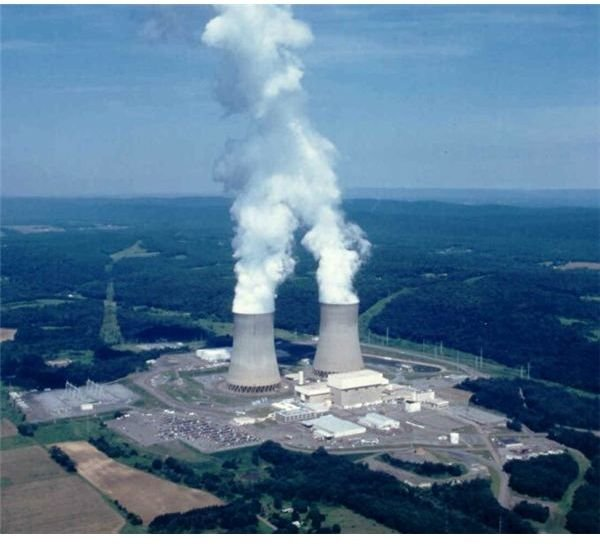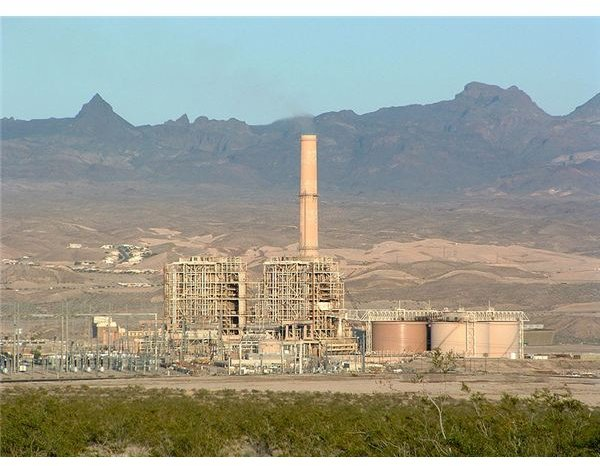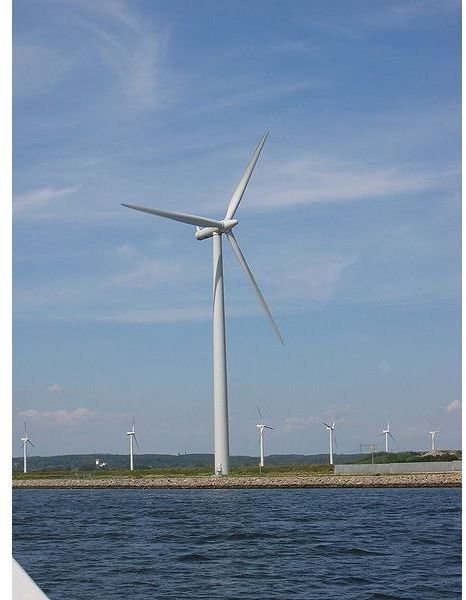## Transmission of Power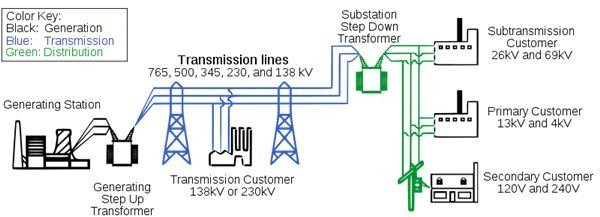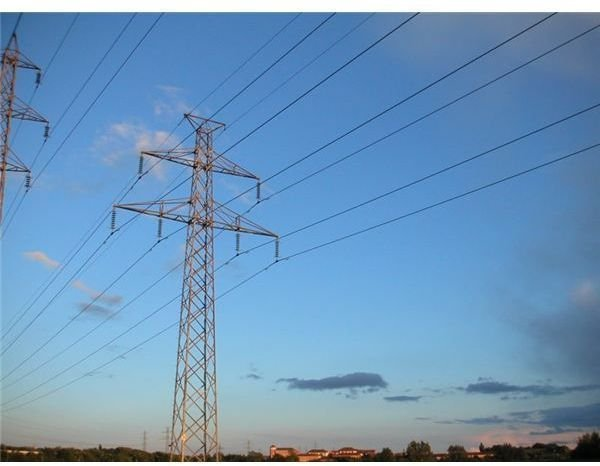## Transmission of Electric Power

Once the power is generated from the alternator, it is send to the typical substation in the power plant where they step up the voltage by using the step-up transformers for transmission purposes.

As the voltage is stepped up, it reduces the transmission losses. It is then sent to the power grid from where it is then transmitted to different cities. All the power generated in different places by different methods is stepped up and sent to a common place called the grid.

Why do we need to step up the voltage for transmission?

For long distance transmission, power lines are made of conducting material like aluminum. There is always some power loss associated with these lines.

If I is the current through the wire and R is the resistance, a considerable amount of electric power (I2R) is dissipated as heat. Hence, the power at the receiving end will be much less than the actual power generated.

However by transmitting the electrical energy at higher voltage, power loss can be controlled as is evident from the following cases:

Case 1:

Power Transmitted At Lower Voltage:

A Power of 11,000 Watts is transmitted at 220 Volt.

Formula for Power (P) = V × I

V-Voltage

I-Current

Therefore, Current (I) =P / V

Current (I) =11,000 / 220.

Current (I) =50 Ampere.

If R is the Resistance of the line wire,

Then the power Loss:

Formula, Power Loss = I2 × R

Power Loss = 502 × R watts = (25000 × R) Watts.

Case 2:

Power Transmitted At Higher Voltage:

If 11,000 Watts power is transmitted at 22,000 Volt

Current (I) =P/V = 11,000/22,000 =0.5 Ampere.

Power loss = I2 × R = (.5)2× R = (0.25 × R ) watts.

From the case 1 and 2, we found that when the power is transmitted at higher voltage, the power losses are less. Hence it is evident that if power is transmitted at a higher voltage, the loss of energy in the form of heat can be considerably reduced.

For transmitting electric power at 11,000W at 220 V the current capacity of line wire has to be 50 A and if transmission is done at 22,000V it is only 0.5 A.

Thus for carrying larger current (50A), thick wires have to be used. This increases the cost of transmission to support these thick wires, and stronger poles have to be erected, which further adds on to the cost. On the other hand, if transmission is done at high voltages, the wires required are of lower current carrying capacity, so thicker wires can be replaced by thin wires, thus reducing the cost of transmission considerably.

An example would be if 400MW power is produced at 15,000V in a power station, it could be stepped up to 230,000V before transmission. The power is then transmitted through the transmission lines or network to the power grid.

## Power Grid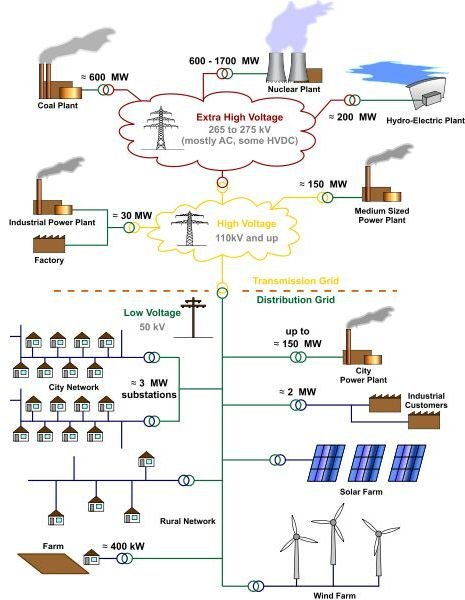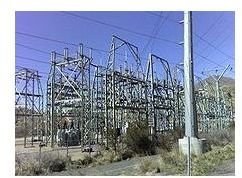## Distribution of Electric Power

The electric power grid connects different parts of the country. And the grid distributes the power to the different parts of the country or state through the transmission lines or network connecting different cities.

Outside the city, the transmitted power from the grid is stepped down in voltage to 110,000 Volt from 230,000 Volt by a step-down auto transformer. Again the power is stepped down to 11,000 Volt from 110,000 Volt by a Step Down power transformer located in the streets and it is distributed to the industrial uses.

Finally before distribution to the domestic user, the power is stepped down to 230 V or 440 V depending upon the needs of the domestic user.

Image Credit:

wikipedia.org

en.wikipedia.org/wiki

## Read More

The Energy Road Map – theenergyroadmap.com

Energy/Ideas/Change – climatechangecentral.com